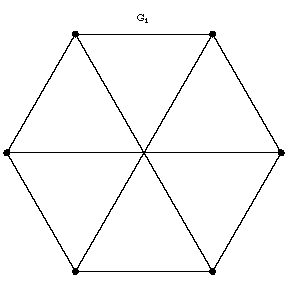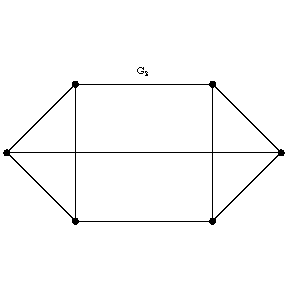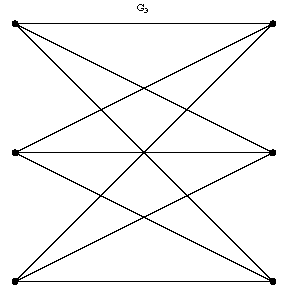# Graph isomorphism

An equivalence relation on the set of graphs. An isomorphic mapping of a non-oriented graph to another one is a one-to-one mapping of the vertices and the edges of one graph onto the vertices and the edges, respectively, of the other, the incidence relation being preserved. Two graphs are said to be isomorphic if there exists an isomorphic mapping of one of these graphs to the other. In the figure, the graphs $G_1$ and $G_2$ are not isomorphic, whereas the graphs $G_1$ and $G_3$ are isomorphic. Isomorphic graphs are usually not distinguished from one another. The number of pairwise non-isomorphic graphs with a given number of vertices and a given number of edges is finite. Isomorphism of oriented graphs, hypergraphs and networks can be defined in a similar manner.Figure: g044950aFigure: g044950bFigure: g044950c

The problem of establishing an isomorphism between graphs is an important problem in graph theory. There are algorithms for certain classes of graphs with the aid of which isomorphism can be fairly effectively recognized (e.g. for trees, cf. Tree, or planar graphs, ). Certain classes of graphs with $n$ vertices have been proved to be uniquely reconstructible (up to an isomorphism) from the set of subgraphs $G-v$ of it with $n-1$ vertices obtained by removing the vertex $v$ in all possible ways. This has been established, in particular, for trees and tournaments (for $n \neq 5,6$, cf. Tournament)

How to Cite This Entry:
Graph isomorphism. Encyclopedia of Mathematics. URL: http://encyclopediaofmath.org/index.php?title=Graph_isomorphism&oldid=33821
This article was adapted from an original article by V.B. Alekseev (originator), which appeared in Encyclopedia of Mathematics - ISBN 1402006098. See original article